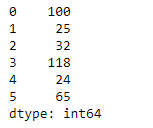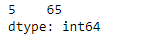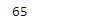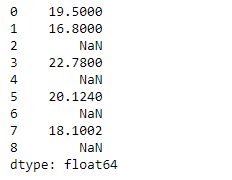Related Articles
Python | Pandas Series.squeeze()
• Last Updated : 05 Feb, 2019

Python is a great language for doing data analysis, primarily because of the fantastic ecosystem of data-centric python packages. Pandas is one of those packages and makes importing and analyzing data much easier.

Pandas series is a One-dimensional ndarray with axis labels. The labels need not be unique but must be a hashable type. The object supports both integer- and label-based indexing and provides a host of methods for performing operations involving the index.

Pandas` Series.squeeze()` function squeeze 1 dimensional axis objects into scalars. Series or DataFrames with a single element are squeezed to a scalar. DataFrames with a single column or a single row are squeezed to a Series. Otherwise the object is unchanged.

Syntax: Series.squeeze(axis=None)

Parameter :
axis : A specific axis to squeeze. By default, all length-1 axes are squeezed.

Returns : Projection after squeezing axis or all the axes.

Example #1 : Use `Series.squeeze()` function to squeeze the single element of the given series to scalar.

 `# importing pandas as pd ` `import` `pandas as pd ` ` `  `# Creating the Series ` `sr ``=` `pd.Series([``100``, ``25``, ``32``, ``118``, ``24``, ``65``]) ` ` `  `# Print the series ` `print``(sr) `

Output :Let’s transform the series in a way that it only contains those elements which are divisible by 13.

 `# Keep only those elements which are divisible by 13 ` `sr_temp ``=` `sr[sr ``%` `13` `=``=` `0``] ` ` `  `# Let's print the series ` `print``(sr_temp) `

Output :Now we will use `Series.squeeze()` function to reduce the given series object to a scalar.

 `# squeeze the series to scalar ` `sr_temp.squeeze() `

Output :As we can see in the output, `Series.squeeze()` function has successfully reduced the given series to a scalar.

Example #2 : Use `Series.squeeze()` function to squeeze the given series object.

 `# importing pandas as pd ` `import` `pandas as pd ` ` `  `# Creating the Series ` `sr ``=` `pd.Series([``19.5``, ``16.8``, ``None``, ``22.78``, ``None``, ``20.124``, ``None``, ``18.1002``, ``None``]) ` ` `  `# Print the series ` `print``(sr) `

Output :Now we will use `Series.std()` function to squeeze the given series object.

 `# squeeze the series to scalar ` `sr_temp.squeeze() `

Output :As we can see in the output, `Series.squeeze()` function has returned the same series object because there are more than one elements in the given series object and hence it could not be squeezed to a scalar value.

Attention geek! Strengthen your foundations with the Python Programming Foundation Course and learn the basics.

To begin with, your interview preparations Enhance your Data Structures concepts with the Python DS Course.

My Personal Notes arrow_drop_up
Recommended Articles
Page :